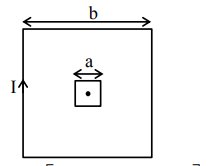# A small square loop of side ' a ' and one turn is placedQuestion:

A small square loop of side ' $a$ ' and one turn is placed inside a larger square loop of side $b$ and one turn $(b>>a)$. The two loops are coplanar with their centres coinciding. If a current $I$ is passed in the square loop of side ' $b$ ', then the coefficient of mutual inductance between the two loops is :

1. $\frac{\mu_{0}}{4 \pi} 8 \sqrt{2} \frac{\mathrm{a}^{2}}{\mathrm{~b}}$

2. $\frac{\mu_{0}}{4 \pi} \frac{8 \sqrt{2}}{a}$

3. $\frac{\mu_{0}}{4 \pi} 8 \sqrt{2} \frac{b^{2}}{a}$

4. $\frac{\mu_{0}}{4 \pi} \frac{8 \sqrt{2}}{b}$

Correct Option: 1

Solution:$\mathrm{B}=\left[\frac{\mu_{0}}{4 \pi} \frac{\mathrm{I}}{\mathrm{b} / 2} \times 2 \sin 45\right] \times 4$

$\phi=2 \sqrt{2} \frac{\mu_{0}}{\pi} \frac{1}{b} \times a^{2}$

$\therefore M=\frac{\phi}{I}=\frac{2 \sqrt{2} \mu_{0} a^{2}}{\pi b}=\frac{\mu_{0}}{4 \pi} 8 \sqrt{2} \frac{a^{2}}{b}$

Option (1)